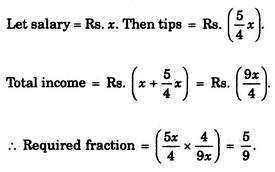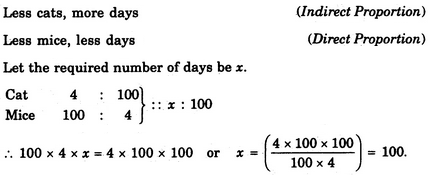# Verbal Reasoning - Arithmetic Reasoning

### Exercise :: Arithmetic Reasoning - Section 1

21.

In a class, there are 18 boys who are over 160 cm tall. If these constitute three-fourths of the boys and the total number of boys is two-thirds of the total number of students in the class, what is the number of girls in the class ?

 A. 6 B. 12 C. 18 D. 24

Explanation:

Let the number of boys be x. Then, (3/4)x = 18 or x = 18 x(4/3) = 24.

If total number of students is y, then (2/3) y = 24 or y = 24 x (3/2) = 36.

Therefore Number of girls in the class = (36 - 24) = 12.

22.

A father is now three times as old as his son. Five years back, he was four times as old as his son. The age of the son (in years) is

 A. 12 B. 15 C. 18 D. 20

Explanation:

Let son's age be x years. Then, father's age = (3x) years.

Five years ago, father's age = (3x - 5) years and son's age = (x - 5) years.

So, 3x - 5 = 4 (x - 5)3x - 5 = 4x - 20x = 15.

23.

A waiter's salary consists of his salary and tips. During one week his tips were 5/4 of his salary. What fraction of his income came from tips ?

 A. 4/9 B. 5/4 C. 5/8 D. 5/9

Explanation:24.

If you write down all the numbers from 1 to 100, then how many times do you write 3 ?

 A. 11 B. 18 C. 20 D. 21

Explanation:

Clearly, from 1 to 100, there are ten numbers with 3 as the unit's digit- 3, 13, 23, 33, 43, 53, 63, 73, 83, 93; and ten numbers with 3 as the ten's digit - 30, 31, 32, 33, 34, 35, 36, 37, 38, 39.

So, required number = 10 + 10 = 20.

25.

If 100 cats kill 100 mice in 100 days, then 4 cats would kill 4 mice in how many days ?

 A. 1 day B. 4 days C. 40 days D. 100 days